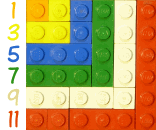# 平方与奇数

• 1 是个平方数（= 1 × 1）
• 1 + 3 = 4，4 是个平方数（= 2 × 2）
• 1 + 3 + 5 = 9，9 是个平方数（= 3 × 3）
• ……！

1 1 = 1 × 1
3 4 = 2 × 2
5 9 = 3 ×3
7 16 = 4 × 4
9 25 = 5 × 5
11 36 = 6 × 6

### 这是魔法吗？• 1 是个正方形（1×1 的正方形）
• 3 就是个 2×2 的正方形
• 5 就是个 3×3 的正方形
• 等等